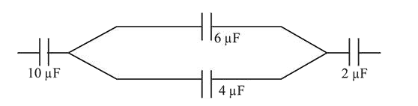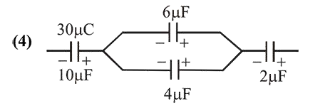# In the figure shown below,

Question:

In the figure shown below, the charge on the left plate of the $10 \mu \mathrm{F}$ capacitor is $-30 \mu \mathrm{C}$. The charge on the right plate of the $6 \mu \mathrm{F}$ capacitor is :1. (1) $-12 \mu \mathrm{C}$

2. (2) $+12 \mu \mathrm{C}$

3. (3) $-18 \mu \mathrm{C}$

4. (4) $+18 \mu \mathrm{C}$

Correct Option: , 4

Solution:As given in the figure, $6 \mu \mathrm{F}$ and $4 \mu \mathrm{F}$ are in parallel. Now using charge conservation\

Charge on $6 \mu \mathrm{F}$ capacitor $=\frac{6}{6+4} \times 30$

$=18 \mu \mathrm{C}$

Since charge is asked on right plate therefore is $+18 \mu \mathrm{C}$